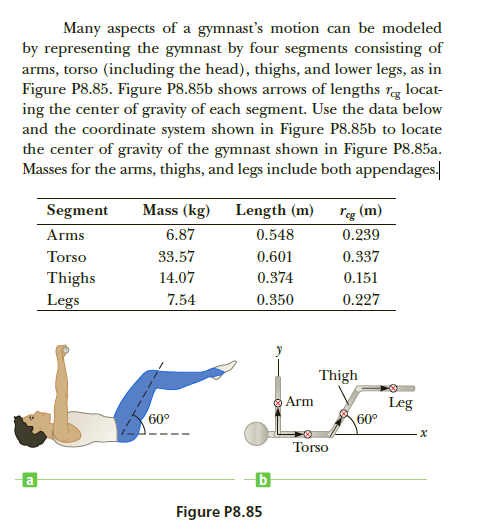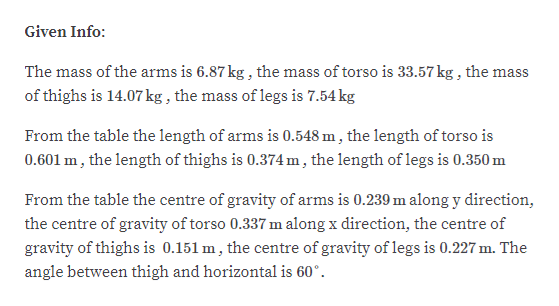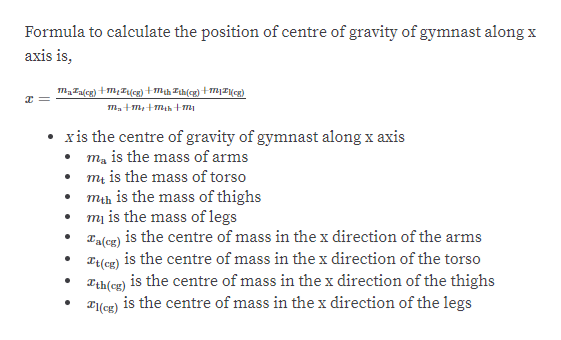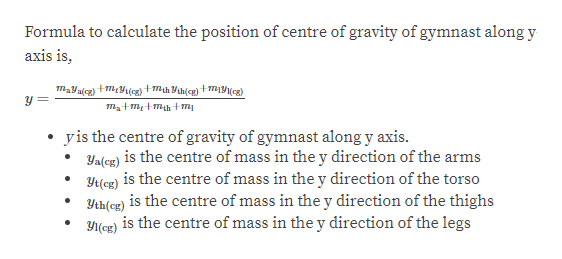# Many aspects of a gymnast's motion can be modeledby representing the gymnast by four segments consisting ofarms, torso (including the head), thighs, and lower legs, as inFigure P8.85. Figure P8.85b shows arrows of lengths reg locat-ing the center of gravity of each segment. Use the data belowand the coordinate system shown in Figure P8.85b to locatethe center of gravity of the gymnast shown in Figure P8.85a.Masses for the arms, thighs, and legs include both appendages.Mass (kg)"eg (m)0.239SegmentLength (m)6.87Arms0.5480.33733.57Torso0.60114.070.374Thighs0.1510.2277.54Legs0.350ThighO ArmLeg60°60°TorsoFigure P8.85

Question
10 viewshelp_outlineImage TranscriptioncloseMany aspects of a gymnast's motion can be modeled by representing the gymnast by four segments consisting of arms, torso (including the head), thighs, and lower legs, as in Figure P8.85. Figure P8.85b shows arrows of lengths reg locat- ing the center of gravity of each segment. Use the data below and the coordinate system shown in Figure P8.85b to locate the center of gravity of the gymnast shown in Figure P8.85a. Masses for the arms, thighs, and legs include both appendages. Mass (kg) "eg (m) 0.239 Segment Length (m) 6.87 Arms 0.548 0.337 33.57 Torso 0.601 14.07 0.374 Thighs 0.151 0.227 7.54 Legs 0.350 Thigh O Arm Leg 60° 60° Torso Figure P8.85 fullscreen
check_circle

Step 1help_outlineImage TranscriptioncloseGiven Info: The mass of the arms is 6.87 kg , the mass of torso is 33.57 kg , the mass of thighs is 14.07 kg , the mass of legs is 7.54 kg From the table the length of arms is 0.548 m , the length of torso is 0.601 m, the length of thighs is 0.374 m , the length of legs is 0.350 m From the table the centre of gravity of arms is 0.239 m along y direction, the centre of gravity of torso 0.337 m along x direction, the centre of gravity of thighs is 0.151 m, the centre of gravity of legs is 0.227 m. The angle between thigh and horizontal is 60°. fullscreen
Step 2help_outlineImage TranscriptioncloseFormula to calculate the position of centre of gravity of gymnast along x axis is, maTalcg) +m, Ilcg) +mth Tth(cg)+mI{cg) ma+m,+mih +m xis the centre of gravity of gymnast along x axis ma is the mass of arms mų is the mass of torso mih is the mass of thighs mị is the mass of legs Talcg) is the centre of mass in the x direction of the arms T(cg) is the centre of mass in the x direction of the torso is the centre of mass in the x direction of the thighs Tth(cg) is the centre of mass in the x direction of the legs T(cg) fullscreen
Step 3help_outlineImage TranscriptioncloseFormula to calculate the position of centre of gravity of gymnast along y axis is, mayaicg) +mYlcx) +mth Yth(cg)+m11(cg) yis the centre of gravity of gymnast along y axis. Ya(cg) is the centre of mass in the y direction of the arms Yr(cg) is the centre of mass in the y direction of the torso Yth(cg) is the centre of mass in the y direction of the thighs is the centre of mass in the y direction of the legs Y1(cg) fullscreen

### Want to see the full answer?

See Solution

#### Want to see this answer and more?

Solutions are written by subject experts who are available 24/7. Questions are typically answered within 1 hour.*

See Solution
*Response times may vary by subject and question.
Tagged in

### Centre of Mass and Centre of Gravity